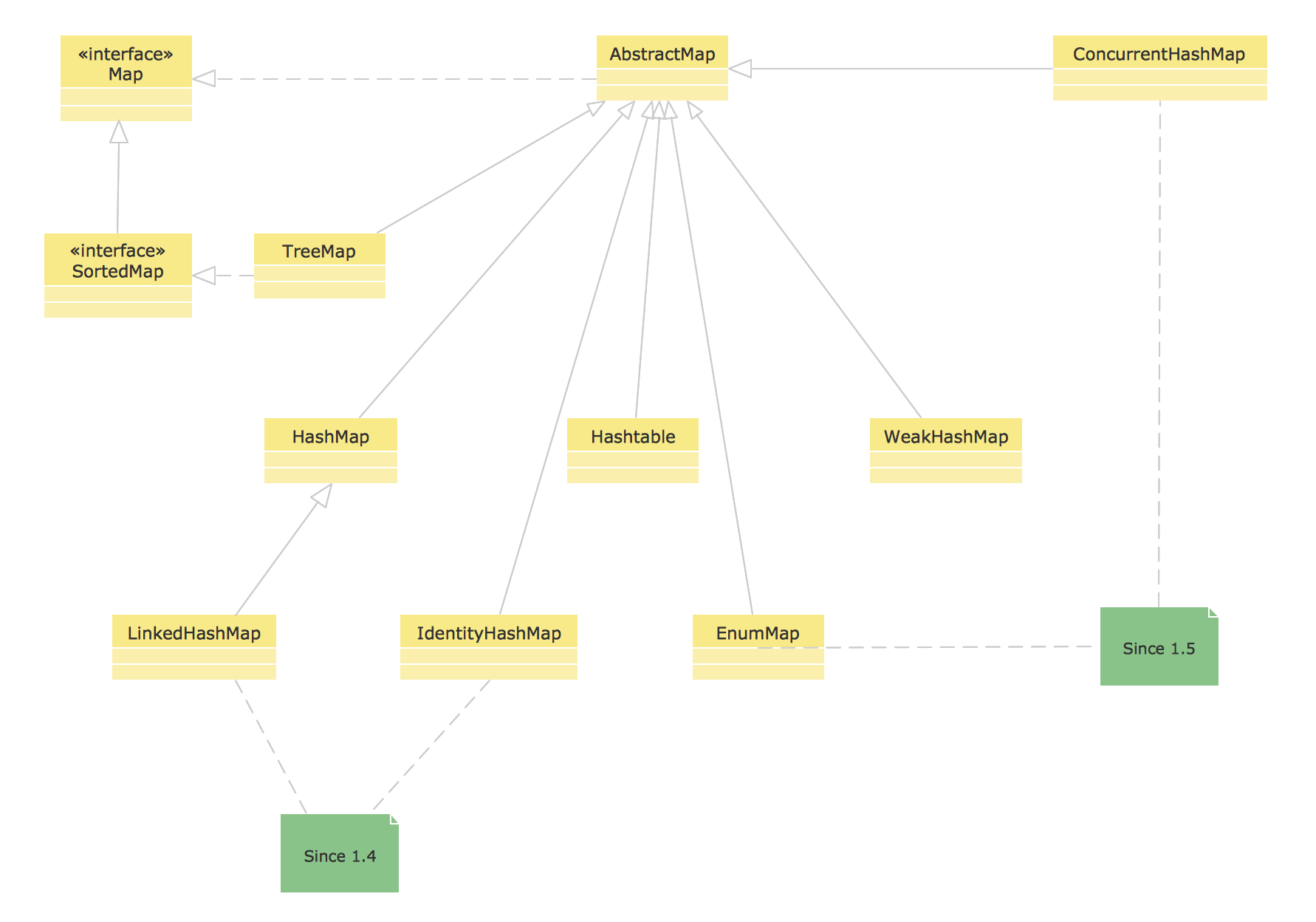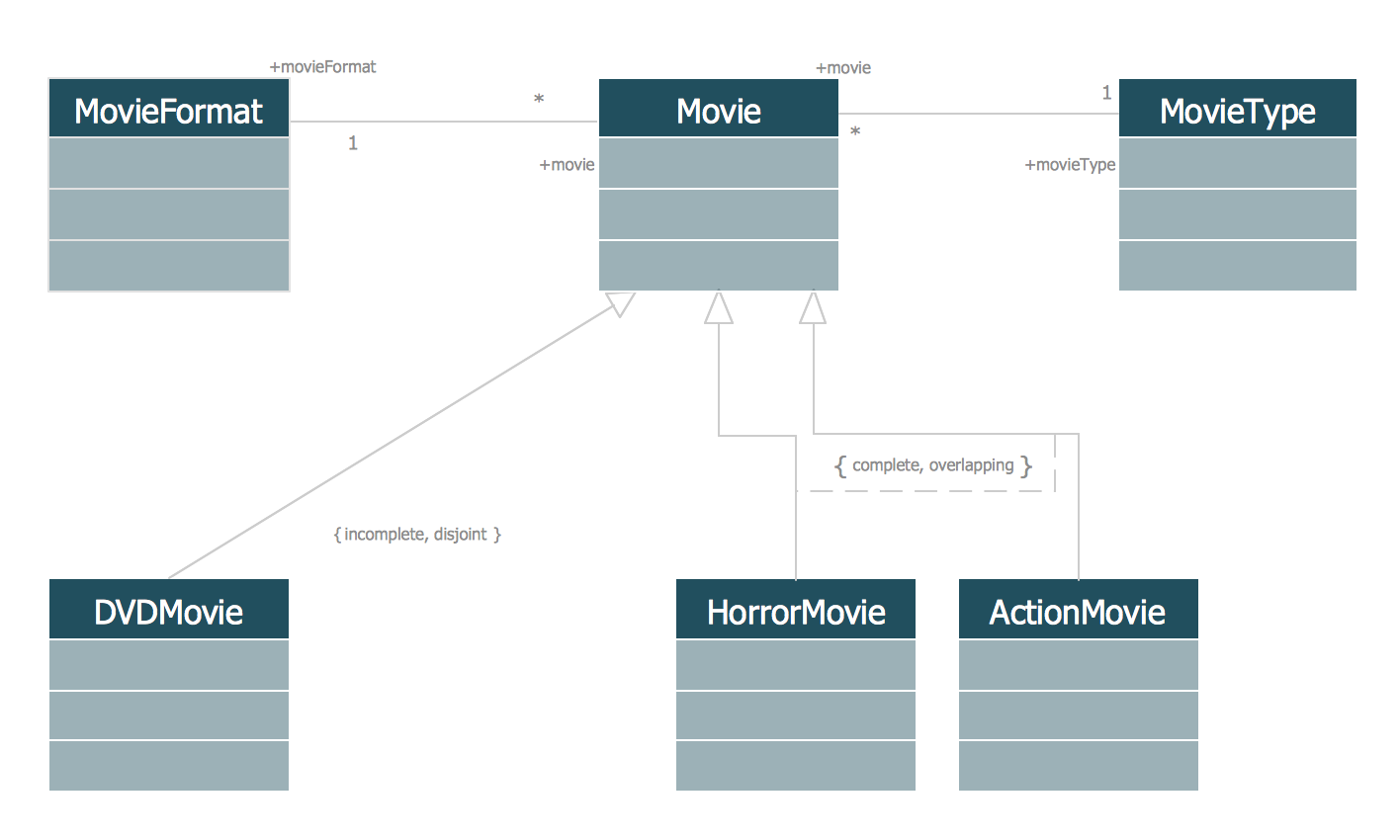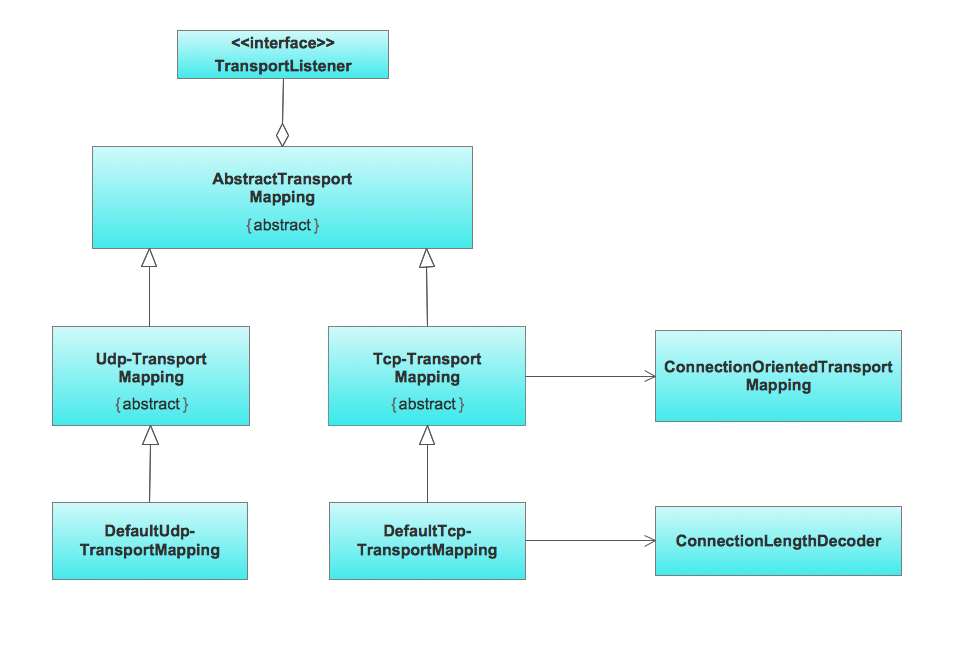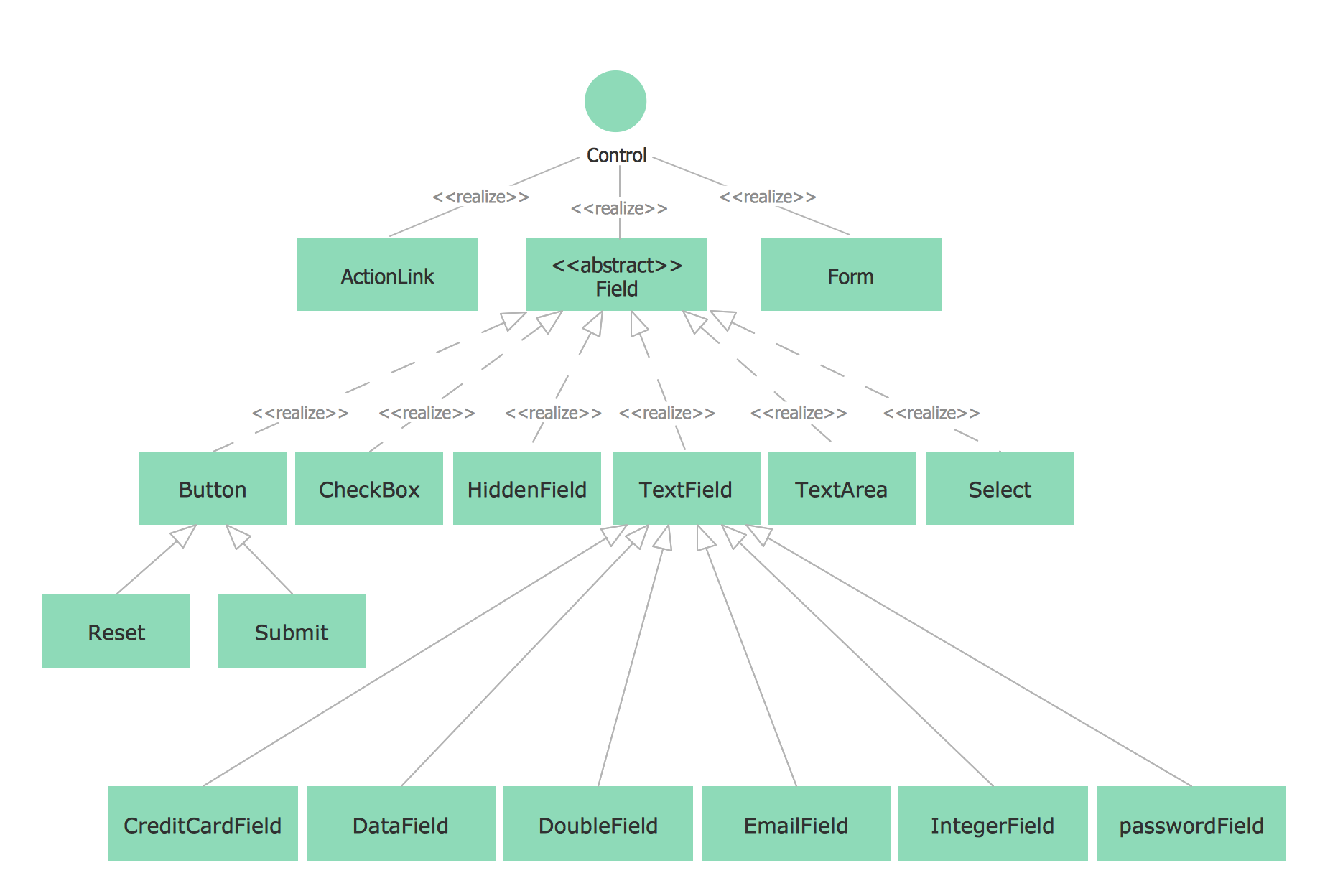ConceptDraw
DIAGRAM 16

# UML Diagram Types

This sample was created in ConceptDraw DIAGRAM diagramming and vector drawing software using the UML Class Diagram library of the Rapid UML Solution from the Software Development area of ConceptDraw Solution Park.

This sample shows the UML Class Diagram of Java map interfaces and implementations. On this diagram you can see the classes represented as boxes and connected with inheritance and realization associations. This sample can be used in Java programming and in education of programmers.Example 1. UML Diagram Types

Using the libraries of the Rapid UML Solution for ConceptDraw DIAGRAM you can create your own visual vector UML diagrams quick and easy.

## UML Resource:

### UML Class Diagram Generalization ExampleUML Diagrams →

This sample was created in ConceptDraw DIAGRAM diagramming and vector drawing software using the UML Class Diagram library of the Rapid UML Solution from the Software Development area of ConceptDraw Solution Park. This sample describes the use of the classes, the generalization associations between them, the multiplicity of associations and constraints. Provided UML diagram is one of the examples set that are part of Rapid UML solution.Picture: UML Class Diagram Generalization ExampleUML Diagrams
Related Solution:

### UML Class Diagram Example for Transport System →

This sample was created in ConceptDraw DIAGRAM diagramming and vector drawing software using the UML Class Diagram library of the Rapid UML Solution from the Software Development area of ConceptDraw Solution Park. This sample shows the transport protocol mappings for SNMP (Simple Network Management Protocol), the classes of the transport system and relationships between them and is used in IP network.Picture: UML Class Diagram Example for Transport System
Related Solution:

### UML Class Diagram Notation →

When it comes to system construction, a class diagram is the most widely used diagram. UML Class Diagrams is a type of static structure diagram that is used for general conceptual modeling of the systematics of the application. Such a diagram would illustrate the object-oriented view of a system. The object orientation of a system is indicated by a class diagram. It describes the structure of a system by showing the general issues,classes of a system, attributes, operations, interfaces, inheritance, and the relationships between them.Picture: UML Class Diagram Notation
Related Solution:
ConceptDraw
DIAGRAM 16